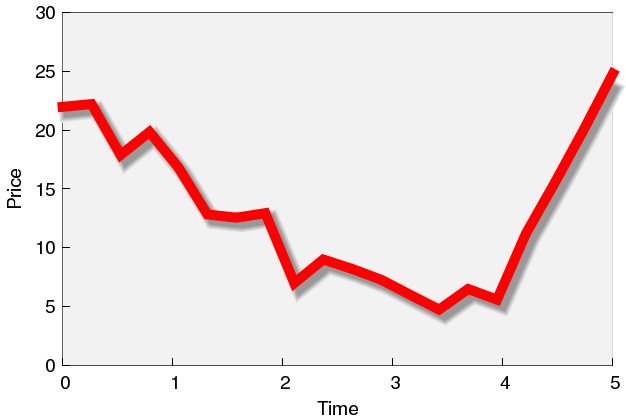In this section, I will discuss how we can give the impression that a 2D plot is in 3D, simply by adding a slight shadow to a curve. Here is the figure that we will haveand here is the script that produced the image

```reset
h(x) = x*x*x-5*x*x+x+20
a = 0.05
b-0.5
unset colorbox

set iso 2, 2
set table 'thickbg.dat'
sp [0:5] [0:30] y
unset table

set sample 20
set table 'thick.dat'
plot [0:5] h(x)+5*(0.5-rand(0))
unset table

set xlabel '{/Helvetica=14 Time}
set ylabel '{/Helvetica=14 Price}'
set tics font "Helvetica, 14" nomirror
set grid front
unset grid
set palette defined (0 0.95 0.95 0.95, 1 0.95 0.95 0.95)
plot [0:5] [0:30] 'thickbg.dat' w ima t '',\
'thick.dat' u (\$1+a):(\$2+b) w l lc rgb "#eeeeee" lw 19 t '',\
'' u (\$1+a):(\$2+b) w l lc rgb "#dddddd" lw 17 t '',\
'' u (\$1+a):(\$2+b) w l lc rgb "#cccccc" lw 15 t '',\
'' u (\$1+a):(\$2+b) w l lc rgb "#bbbbbb" lw 13 t '',\
'' u (\$1+a):(\$2+b) w l lc rgb "#aaaaaa" lw 11 t '',\
'' u (\$1+a):(\$2+b) w l lc rgb "#999999" lw 9 t '',\
'' u 1:2 w l lc rgb "#ff0000" lw 10 t  ''
```

The whole (and very simple) idea is that we will add a replica of the curve that we want to plot to the figure, in some shade of gray, and shifted a bit, so as to give the impression that this curve is the shadow of the other one.

First, we define three functions. h(x) will generate some data (if you have a data file to plot, then obviously, you can skip this, and the set table... plot h(x) ... unset table section), and a and b define the shift in the horizontal and vertical directions, respectively. We also add a background to the figure, in gray. In order to do so, we splot y (or anything else, for that matter) to a file, and in our real plot, we plot this as an image, with the defined colour palette.

There is some subtlety to the lines

```set grid front
unset grid
```

At first, this appears silly to set something and then unset it immediately afterwards, but there is a very good reason for that. Odd as it might sound, the placement of the tics is defined by set grid, i.e., if we want visible tics on the background, we have to push them to the front, otherwise, the background of our figure would cover them. We do this by setting grid front. However, we do not actually want any grid lines, so we unset them. The effect on the tics remains, however. The last step before plotting our curve is to plot the shadow. We do it in 6 steps, and in each step we reduce line width and the whiteness of the colour. If you look at the colour definitions, consecutive lines are in darker shades of gray. The reason for this is that in this way the edge of the shadow will not be sharp. However, if you are satisfied with a sharp shadow, you can skip 5 out of these 6 steps, and draw the shadow only once.